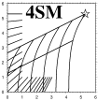LANDSAT 8 OLIP
A time series of Landsat 8 OLIP images
at Lizard Island, GBR, Australia

work done in 2020 at 15 m GSD

 Using the Panchromatic band for water column correction to derive water depth and spectral bottom signature: Landsat 8 OLIP bandset used for this work Purple=1, Blue=2, Green=3, PAN=4, Red=5, NIR=6 and SWIR1=7peer-reviewed and published in 2017home

500*2000 HICO image of Bahrain, october 21rst 2013
scene iss.2013294.1021.093734.L1B.Bahrain.v04.14946.20131021200925.100m.hico courtesy of OSU
HICO: Hyperspectral Imager for the Coastal Ocean: GSD 100 m
work done march 2016

home

# The data

HICO bandset in nanometers for 4SM
WLMin[ 1]=401.2 WL[ 1]=406.9 WLMax[ 1]=412.7
WLMin[ 2]=412.7 WL[ 2]=418.4 WLMax[ 2]=424.1
WLMin[ 3]=424.1 WL[ 3]=429.9 WLMax[ 3]=435.6
WLMin[ 4]=435.6 WL[ 4]=441.3 WLMax[ 4]=447.0
WLMin[ 5]=447.1 WL[ 5]=452.8 WLMax[ 5]=458.5
WLMin[ 6]=458.5 WL[ 6]=464.2 WLMax[ 6]=469.9
WLMin[ 7]=470.0 WL[ 7]=475.7 WLMax[ 7]=481.4    BLUE
WLMin[ 8]=481.4 WL[ 8]=487.1 WLMax[ 8]=492.9
WLMin[ 9]=492.9 WL[ 9]=498.6 WLMax[ 9]=504.3
WLMin=504.3 WL=510.0 WLMax=515.8
WLMin=515.8 WL=521.5 WLMax=527.2
WLMin=527.2 WL=533.0 WLMax=538.7
WLMin=538.7 WL=544.4 WLMax=550.1   GREEN
WLMin=550.2 WL=555.9 WLMax=561.6
WLMin=561.6 WL=567.3 WLMax=573.0
WLMin=573.1 WL=578.8 WLMax=584.5
WLMin=584.5 WL=590.2 WLMax=596.0
WLMin=596.0 WL=601.7 WLMax=607.4
WLMin=607.4 WL=613.2 WLMax=618.9
WLMin=618.9 WL=624.6 WLMax=630.3
WLMin=630.4 WL=636.1 WLMax=641.8
WLMin=641.8 WL=647.5 WLMax=653.2   RED
WLMin=653.3 WL=659.0 WLMax=664.7
WLMin=664.7 WL=670.4 WLMax=676.1
WLMin=676.2 WL=681.9 WLMax=687.6
WLMin=687.6 WL=693.3 WLMax=699.1
WLMin=699.1 WL=704.8 WLMax=710.5   PAN
WLMin=836.6 WL=842.3 WLMax=848.0   NIR
Data preparation

• Binning: HICO's original bands 1_to_52 are binned pairwise into 26 new_channels
• visible bands are placed in channels 1 to 26 of the working database
• and NIR band is placed into channel_28 of the working database
• this is the maximum number of channels in the 4SM command line, even using a full HD screen!
•
• new_channels 10_to_26 are binned into a panchromatic new_channel
• that's all of the Green-Red region
• this new_channel is placed in channel_27 of the working database
• and assigned a wavelength of 705 nm

•

# Deglinting

• adjacency effect does not dominate the NIR signal
•

• by contrast, sea-surface clutter is a significant source of variation of the NIR signal